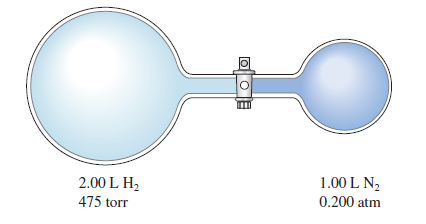# Problem: Consider the flask apparatus below, which now contains 2.00 L H  2 at a pressure of 360. torr and 1.00 L N2 at an unknown pressure. If the total pressure in the flasks is 320. torr after the stopcock is opened, determine the initial pressure of N2 in the 1.00-L flask.

⚠️Our tutors found the solution shown to be helpful for the problem you're searching for. We don't have the exact solution yet.

###### Problem Details

Consider the flask apparatus below, which now contains 2.00 L H  2 at a pressure of 360. torr and 1.00 L N2 at an unknown pressure. If the total pressure in the flasks is 320. torr after the stopcock is opened, determine the initial pressure of N2 in the 1.00-L flask.